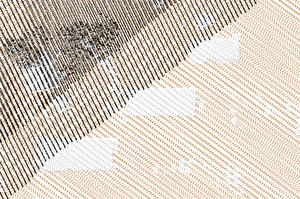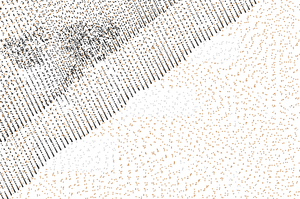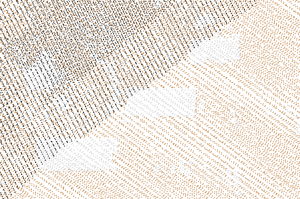## NAME

v.decimate - Decimates a point cloud
Copies points from one vector to another while applying different decimations

## KEYWORDS

vector, LIDAR, generalization, decimation, extract, select, points, level1

## SYNOPSIS

v.decimate
v.decimate --help
v.decimate [-gfczxbt] input=name [layer=string] output=name [zrange=min,max] [cats=range] [skip=integer] [preserve=integer] [offset=integer] [limit=integer] [zdiff=float] [cell_limit=integer] [--overwrite] [--help] [--verbose] [--quiet] [--ui]

### Flags:

-g
Apply grid-based decimation
-f
Use only first point in grid cell during grid-based decimation
-c
Only one point per cat in grid cell
-z
Use z in grid decimation
-x
Store only the coordinates, throw away categories
Do not story any categories even if they are present in input data
-b
Do not build topology
Advantageous when handling a large number of points
-t
Do not create attribute table
--overwrite
Allow output files to overwrite existing files
--help
Print usage summary
--verbose
Verbose module output
--quiet
Quiet module output
--ui
Force launching GUI dialog

### Parameters:

input=name [required]
Name of input vector map
Or data source for direct OGR access
layer=string
Layer number or name ('-1' for all layers)
A single vector map can be connected to multiple database tables. This number determines which table to use. When used with direct OGR access this is the layer name.
Default: -1
output=name [required]
Name for output vector map
zrange=min,max
Filter range for z data (min,max)
cats=range
Category values
Example: 1,3,7-9,13
skip=integer
Throw away every n-th point
For example, 5 will import 80 percent of points. If not specified, all points are copied
preserve=integer
Preserve only every n-th point
For example, 4 will import 25 percent of points. If not specified, all points are copied
offset=integer
Skip first n points
Skips the given number of points at the beginning.
limit=integer
Copy only n points
Copies only the given number of points
zdiff=float
Minimal difference of z values
Minimal difference between z values in grid-based decimation
cell_limit=integer
Preserve only n points per grid cell
Preserves only the given number of points per grid cell in grid-based decimation

## DESCRIPTION

v.decimate reduces number of points in the input vector map and copies them over to the output vector map. Different point decimation techniques can be applied to reduce the number of points.

Two main decimation techniques are:

• count-based decimation (skip, preserve, offset and limit options)
• grid-based decimation (-g flag)

The grid-based decimation will remove points based on:

• similar z coordinates (-z flag and zdiff option)
• same categories (-c flag)
• count of points (-f flag and cell_limit option)

The grid-based decimation is currently using a 2D grid, so the points are placed and compared within this 2D grid. The comparison can happen using z coordinates or categories. Note that although the grid is only 2D, the module works with 3D points.

The grid-based decimation extent and resolution depend on the current computational region as set by g.region. As a consequence, the output is limited only to computational region in this case.

TODO: Currently, any output is limited by the region.

The count-based decimation result highly depends on how the data are ordered in the input. This applies especially to offset and limit options where the resulting shape and densities can be surprising. The options skip and preserve are influenced by order of points in a similar way but they usually keep relative density of points (which may or may not be desired). On the other hand, the grid-based decimation will generally result in more even density of output points (see Figure 1).

Besides decimation, point count can be reduced by applying different selections or filters, these are:

• selection by category (cats option)
• selection by z values (zrange option)

## NOTES

The grid-based decimation requires all points which will be saved in output to fit into the computer's memory (RAM). It is advantageous to have the region only in the area with the points, otherwise unnecessary memory is allocated. Higher (finer) resolutions and higher amount of preserved points per cell require more memory. The count-based decimation has no limitation regarding the available memory.

Significant speed up can be gained using -b flag which disables building of topology for the output vector map. This may limit the use of the vector map by some modules, but for example, this module works without topology as well.

## EXAMPLES

Keep only every forth point, throw away the rest:
```v.decimate input=points_all output=points_decimated_every_4 preserve=4
```

Keep only points within a grid cell (given by the current computational region) which has unique categories (e.g. LIDAR classes):

```v.decimate input=points_all output=points_decimated_unique_cats layer=1 -g -c
```Figure 1: Comparison of original points, decimation result with every forth point preserved, and grid-based decimation result with points with unique categories in each grid cell

Keep only points with category 2 and keep only approximately 80% of the points:

```v.decimate input=points_all output=points_decimated_ skip=5 cats=2 layer=1
```

## REFERENCES

• Petras, V., Petrasova, A., Jeziorska, J., Mitasova, H. (2016). Processing UAV and LiDAR point clouds in grass GIS. The International Archives of Photogrammetry, Remote Sensing and Spatial Information Sciences, 41, 945 (DOI)